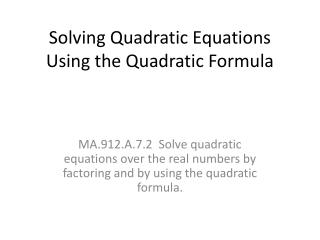DownloadDownload PresentationDownload Presentation- - - - - - - - - - - - - - - - - - - - - - - - - - - E N D - - - - - - - - - - - - - - - - - - - - - - - - - - -
##### Presentation Transcript

1. Solving Quadratic Equations Using the Quadratic Formula MA.912.A.7.2 Solve quadratic equations over the real numbers by factoring and by using the quadratic formula.

2. The Quadratic Formula The solutions of a quadratic equation written in Standard Form, ax2 + bx + c = 0, can be found by Using the Quadratic Formula. Click on the link below to view a song to help you memorize it. http://www.regentsprep.org/Regents/math/algtrig/ATE3/quadsongs.htm

3. Deriving the Quadratic Formula by Completing the Square. Divide both sides by “a”. Subtract constant from both sides.

4. Deriving the Quadratic Formula by Completing the Square. Complete The Square Factor the Perfect Square Trinomial Simplify expression on the left side by finding the LCD

5. Deriving the Quadratic Formula by Completing the Square. Take the square root of both sides Solve absolute value/ Simplify radical

6. Deriving the Quadratic Formula by Completing the Square. Isolate x Simplify Congratulations! You have derived The Quadratic Formula

7. #1 Solve using the quadratic formula.

9. #1 Solve by factoring

10. #2 Solve by factoring This quadratic is Prime (will not factor), The Quadratic Formula must be used!

11. #2 Solve using the quadratic formula. Exact Solution Approx Solution

13. #3 Solve using the quadratic formula The is not a real number, therefore this equation has ‘NO Real Solution’

15. #4 Solve using the quadratic formula Would factoring work to solve this equation?

17. #5 Solve using the quadratic formula. Exact Solution Approx Solution

18. #5 What if we move everything to the right side? Exact Solution Approx Solution